Next: Inclusion of Geometrical Constraints Up: Vertex Fitting Previous: Vertex Fitting without Error

## Vertex Fitting with Error Matrix

When error matrices are available, the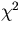to minimize is defined by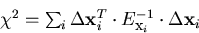(33)
where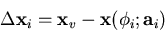and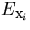is the position error matrix of i-th track at the deflection angle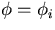corresponding to the trial vertex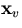: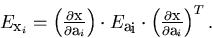The parameter vector to determine byminimization is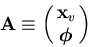where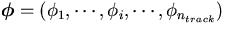and thus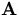contains 3 + nn<<848>>track parameters. Theminimization requires the calculations of the first and the second derivatives of the.The first derivatives are calculated as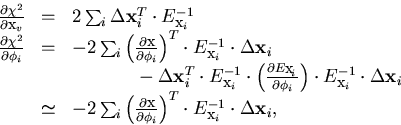(34)
where in the last step we have ignored the second order term with respect to the residual vector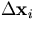which is a good approximation near theminimum. From these the second derivatives are readily obtained: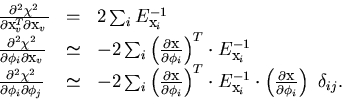(35)
Notice that when the position error matrices are unity, the problem is equivalent to the one in the last subsection. The difference here is the introduction of the error matrices as metric tensors in the residual space.

Once the derivatives are calculated, all we have to do is to solve the following linear equation (multi-dimensonal Newton's method) iteratively: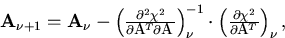(36)
where the diagonal elements of the second derivative matrix should be multiplied by some number greater than one when theincreases as with the track fitting in Section 2.2.

When the fit converges, the error matrix for the parameter vector is obtained to be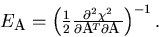(37)Next: Inclusion of Geometrical Constraints Up: Vertex Fitting Previous: Vertex Fitting without Error
Keisuke Fujii
12/4/1998